SEARCH HOMEMath Central Quandaries & QueriesQuestion from Rajesh, a student: A square piece of a cardboard of sides ten inches is taken aND FOUR equal peices are removed at the corner. Then sides are turned up to form an open box then the maximum volume such a box can have.Hi Rajesh.

I am sure you have recognized that a very thin flat box will not hold much volume, and neither will a very tall box with a small base. There must be some value between the extremes that maximizes the volume of the box.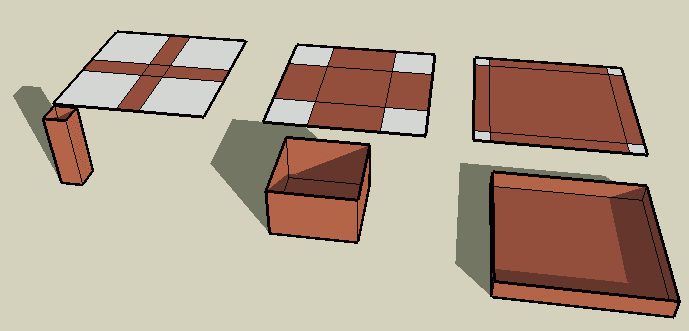Let's consider this algebraically: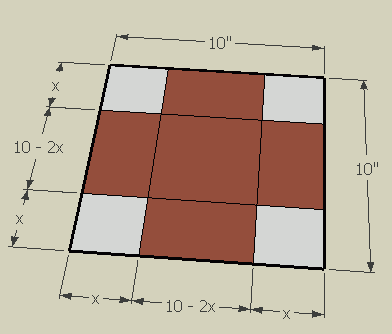So the volume of the box is its length times width times depth: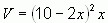Now take the derivative of both sides of this equation with respect to the variable x: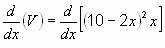You will need to use the chain rule to solve this. Remember that a really narrow box has a small volume, as does a really thin box, but in between these extremes, we find a maximum volume. This is a critical point, so the derivative at the this point is zero. Thus, all you need to do is solve for x by making dV/dx = 0: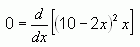Solve for x.

Cheers,
Stephen La Rocque.Math Central is supported by the University of Regina and The Pacific Institute for the Mathematical Sciences.